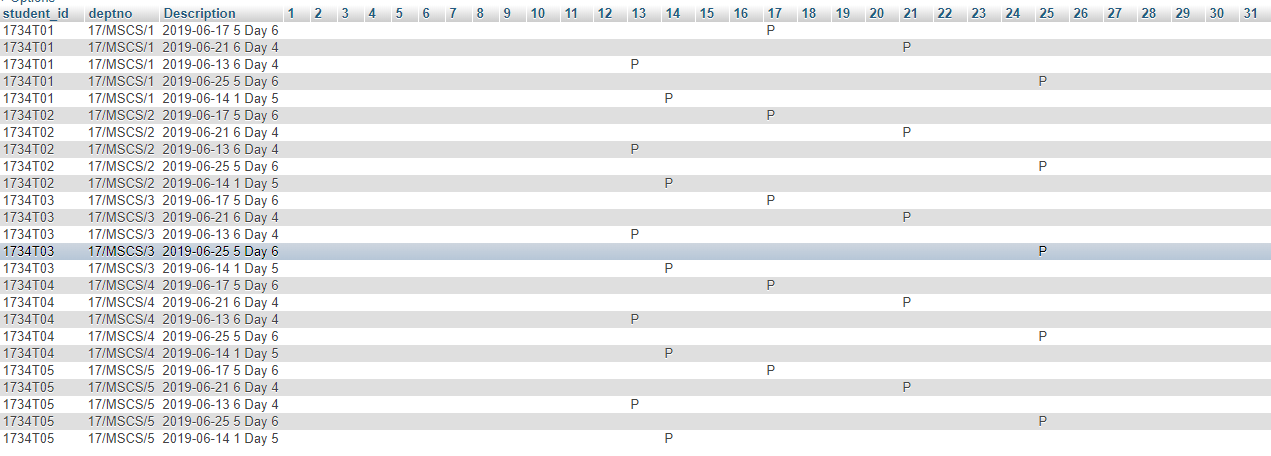# #StackBounty: #mysql #pivot #dynamic-sql #phpmyadmin Monthly Date and Hour wise report generation using PHP MYSQL

### Bounty: 50

Attendance Portal in PHP

I am facing problem at Final Attendance Report Generation

I’m stuck at the final stage of the Project:

I have a Table- attendance, If a student is Present I mark it as 1 if absent 0. we have a date, day order, hour (we are following Day Order Time Table)

`````` Table
dayorder:
id day
1 day1
2 day2
3 day3
4 day4
5 day5
6 SAT
7 day6
Table
Hour:
id
1
2
3
4
5
6
7
``````

I must generate a Monthly report like a register as belowCode I have Tried:

``````SELECT student_id,deptno,Month, Year_c,
branch.description as bdesc,
course.coursecode as ccd,
users.firstname as ufn,
users.lastname as uln,
course.description as ccdes,schedules.hour as hhour,
h,dayorder,AttdDate,
CONCAT(AttdDate,h,dayorder) AS fate,
IF(Day_c=1, p, " ") AS '1',
IF(Day_c=2, p, " ") AS '2',
IF(Day_c=3, p, " ") AS '3',
IF(Day_c=4, p, " ") AS '4',
IF(Day_c=5, p, " ") AS '5',
IF(Day_c=6, p, " ") AS '6',
IF(Day_c=7, p, " ") AS '7',
IF(Day_c=8, p, " ") AS '8',
IF(Day_c=9, p, " ") AS '9',
IF(Day_c=10, p, " ") AS '10',
IF(Day_c=11, p, " ") AS '11',
IF(Day_c=12, p, " ") AS '12',
IF(Day_c=13, p, " ") AS '13',
IF(Day_c=14, p, " ") AS '14',
IF(Day_c=15, p, " ") AS '15',
IF(Day_c=16, p, " ") AS '16',
IF(Day_c=17, p, " ") AS '17',
IF(Day_c=18, p, " ") AS '18',
IF(Day_c=19, p, " ") AS '19',
IF(Day_c=20, p, " ") AS '20',
IF(Day_c=21, p, " ") AS '21',
IF(Day_c=22, p, " ") AS '22',
IF(Day_c=23, p, " ") AS '23',
IF(Day_c=24, p, " ") AS '24',
IF(Day_c=25, p, " ") AS '25',
IF(Day_c=26, p, " ") AS '26',
IF(Day_c=27, p, " ") AS '27',
IF(Day_c=28, p, " ") AS '28',
IF(Day_c=29, p, " ") AS '29',
IF(Day_c=30, p, " ") AS '30',
IF(Day_c=31, p, " ") AS '31'
FROM
(SELECT *,DAY(date) AS Day_c,
MONTHNAME(date) AS Month,
Year(date) AS Year_c,
date(date) AS AttdDate,hour as h, day as dayorder,
(CASE  WHEN present = 1
THEN 'P'
WHEN present = 0
THEN 'A'
WHEN present is null
THEN ' '
END) AS p
FROM attendance a
WHERE date between '\$from' AND '\$to' And branch = \$branchid AND coursecode = \$courseid AND batch = \$batchid
GROUP BY student_id
ORDER BY student_rollno ASC
)
as report
LEFT JOIN branch on branch.id = report.branch
LEFT JOIN course on course.id = report.coursecode